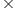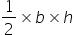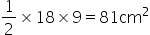Maths-
General
Easy

Question

# Find the area of largest triangle that can be inscribed in a semi-circle of radius 9 cm.Hint:

## The correct answer is: 81 cm2

### It is given that radius of the circle = 9 cm⇒ Diameter = 2r = 29 = 18 cmAs we can see in the pictureBase of the triangle = diameter = 18 cmHeight of the triangle = radius = 9 cmArea of the triangle ==#### With Turito Foundation.#### Get an Expert Advice From Turito.